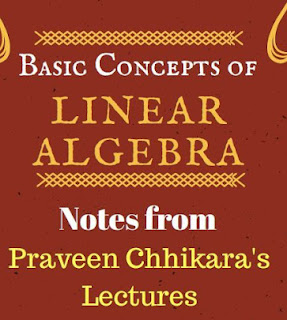# BASIC CONCEPTS OF LINEAR ALGEBRA NOTES

Linear algebra is the branch of mathematics concerning vector spaces and linear mappings between such spaces. It includes the study of lines, planes, and subspaces, but is also concerned with properties common to all vector spaces.PAGE:- 225
The set of points with coordinates that satisfy a linear equation forms a hyperplane in an n-dimensional space. The conditions under which a set of n hyperplanes intersect in a single point is an important focus of study in linear algebra. Such an investigation is initially motivated by a system of linear equations containing several unknowns. Such equations are naturally represented using the formalism of matrices and vectors.

MATRIX HAND WRITTEN NOTE BY PI AIM

Linear algebra is central to both pure and applied mathematics. For instance, abstract algebra arises by relaxing the axioms of a vector space, leading to a number of generalizations. Functional analysis studies the infinite-dimensional version of the theory of vector spaces. Combined with calculus, linear algebra facilitates the solution of linear systems of differential equations.

PARTIAL DIFFERENTIAL EQUATIONS BY RISING STAR ACADEMY

Techniques from linear algebra are also used in analytic geometry, engineering, physics, natural sciences, computer science, computer animation, advanced facial recognition algorithms and the social sciences (particularly in economics). Because linear algebra is such a well-developed theory, nonlinear mathematical models are sometimes approximated by linear models.

NOTE: jigssolanki.in does not own this book, neither created nor scanned. We just providing the link already available on internet. If any way it violates the law or has any issues then kindly mail us: jigssolanki1995@gmail.com or Contact Us for this(Link Removal) issue.